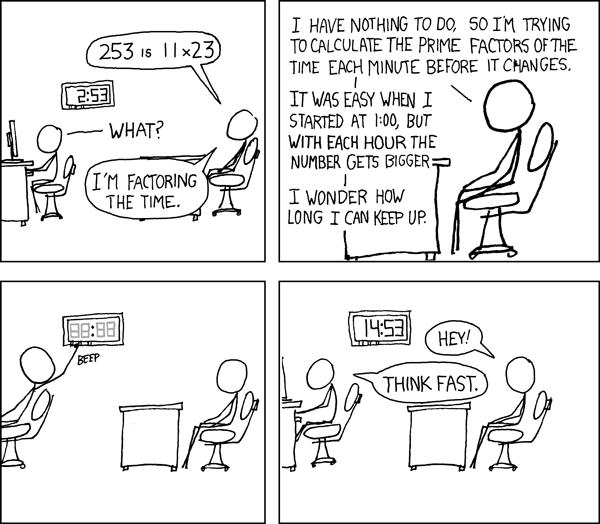# Some Prime Thoughts

Friday, January 5, 2018

The Fundamental Theorem of Arithmetic states that every positive integer can be factored into primes in a unique way.

But first, what is a prime number? A prime number is a number greater than 1 that has no positive divisiors except 1 and itself e.g. 2 is prime. The primality of a given number $$n$$ can be find out by trial and error. It consists of testing whether $$n$$ is a multiple of any integer between $$2$$ and $$\sqrt n$$.

Some better algorithms exist to test the primality of a number.

Coming back to The Fundamental Theorem of Arithmetic, $$98$$ can be written as $$2\,.\,7^2$$. There is no other way to factor it.## Infiniteness of primes

There are infinite prime numbers. One of the earliest proofs was given by Euclid.
Suppose there are finitely many primes. Let’s call them $$p_{1}, p_{2}, p_{3}, ..., p_{k}$$.
Now, consider the number $$p* = p_{1}\,.p_{2}\,...p_{k-1}.p_{k} + 1$$.
$$p*$$ is either prime or not. If it’s prime, then it was not in our list. If it’s not prime, then it’s divisible by some prime, $$p$$. Notice, $$p$$ can’t be $$p_{1}, p_{2}, p_{3}, ..., p_{k}$$ because of remainder 1. Thus, $$p$$ was not in our list. Either way, our assumption that there are finite number of primes is wrong. Hence, there are infinite primes.

## The Quest of larger primes

One of the widely used applications of primes is in the public-key cryptography e.g. in the RSA cryptosystem. The RSA algorithm is based on the concept of trapdoor, a one-way function. Its strength lies in the fact that it’s computationally hard (impossible) to factorize large numbers. For now, there doesn’t exists any efficient algorithm for prime factorization.

For example, It’s easy for a computer to multiply two large prime numbers. But let’s say you multiply two large prime numbers together to get a resulting number. Now, if you give this new number to a computer and try to factorize, the computer will struggle e.g. to find which two prime nubmers are multiplied together to get 18848997161 is difficult.

How can so much of the formal and systematic edifice of mathematics, the science of pattern and rule and order per se, rest on such a patternless, unruly, and disorderly foundation? Or how can numbers regulate so many aspects of our physical world and let us predict some of them when they themselves are so unpredictable and appear to be governed by nothing but chance?” — H. Peter Aleff

The thirst to find the order in primes is one of the reasons for its quest.

References: